### Home > GC > Chapter 12 > Lesson 12.2.4 > Problem12-92

12-92.
1. the diagrams below. For each one, use the geometric relationships to solve for the given variable. Homework Help ✎

1.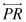is tangent to ⊙C at P and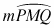= 314°. Find QR.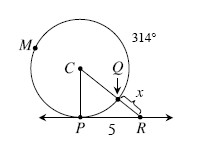2.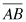and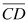intersect at E.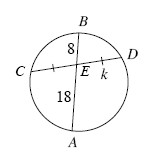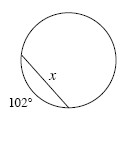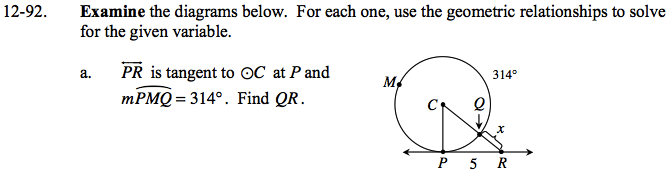$\angle PCQ = 360 - 314 = 46^\circ$

$\text{tan}46^\circ = \frac{5}{\overline{CP}}$

$\overline{CP} = \overline{CQ} \approx 4.83$

$\text{sin}46^\circ = \frac{5}{\overline{CR}}$

$\overline{CR} \approx 6.95$

x ≈ 6.95 − 4.83 ≈ 2.12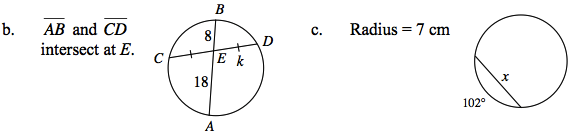(CE)(ED) = (AE)(EB)

(k)(k) = (18)(8)

Draw ΔABC out of the chord that has been given.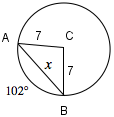According to the Law of Cosines:

$\overline{AB}^2 = \overline{AC}^2 + \overline{BC}^2 - 2 (\overline{AC}) (\overline{BC}) \text{cos}102^\circ$

$x^2 = 7^2 + 7^2 - 2(7)(7)\text{cos}102^\circ$